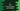# C program to find if two numbers are Amicable or not### C program to find if two numbers are Amicable or not :

Two numbers are known as Amicable numbers if the sum of proper divisors of one number is equal to the other number. In this tutorial, we will check how to find if two numbers are Amicable or not using c programming language. The algorithm we are going to use is as below :

### Algorithm :

1. First ask the user to enter both numbers.
2. Find the sum of all divisors for both numbers.
3. Finally check if the sum of the divisors of one number is equal to the other number or not.
4. If yes, it is a Amicable number and otherwise not.

### C program :

``````#include<stdio.h>

int main()
{
//1
int i;
int firstNumber,secondNumber;

//2
int firstDivisorSum = 0;
int secondDivisorSum = 0;

//3
printf("Enter two numbers to check if Amicable or not : ");
scanf("%d %d",&firstNumber,&secondNumber);

//4
for(int i=1;i<firstNumber;i++){
//5
if(firstNumber % i == 0){
firstDivisorSum = firstDivisorSum + i;
}
}

//6
for(int i=1;i<secondNumber;i++){
if(secondNumber % i == 0){
secondDivisorSum = secondDivisorSum + i;
}
}

//7
if((firstNumber == secondDivisorSum) && (secondNumber == firstDivisorSum)){
printf("%d and %d are Amicable numbers\n",firstNumber,secondNumber);
}else{
printf("%d and %d are not Amicable numbers\n",firstNumber,secondNumber);
}

}
``````

### Explanation :

The commented numbers in the above program denotes the steps below :

1. Create one integer i to use in the loops and create two more integer variables firstNumber , secondNumber to store the first and second number.
2. Create two more integer variables to store the sum of the divisors : firstDivisorSum and secondDivisorSum.
3. Ask the user to input both numbers. Store the values in firstNumber and secondNumber.
4. Use one for loop and check for each numbers starting from 1 to fistNumber -1.
5. Check for each number in the loop if it can divide the number firstNumber or not. If yes, add this number to the firstDivisorSum. After the loop complets, firstDivisorSum contains the sum of all the divisors for firstNumber.
6. Similarly, find the sum of all divisors for the second number and save it in secondDivisorSum.
7. Finally, check if the sum of divisors of one number is equal to the other number or not. If yes, print that both are Amicable numbers. Otherwise they are not.

### Example Output :

``````Enter two numbers to check if Amicable or not : 12 13
12 and 13 are not Amicable numbers

Enter two numbers to check if Amicable or not : 220 284
220 and 284 are Amicable numbers

Enter two numbers to check if Amicable or not : 24 47
24 and 47 are not Amicable numbers

Enter two numbers to check if Amicable or not : 288 822
288 and 822 are not Amicable numbers``````